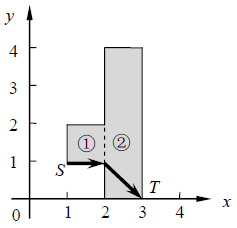## Day 1, Problem 2 - Intelligent Car Racing

The first annual intelligent car competition at JL University has started! The racetrack can be represented as a combination of n rectangular regions (see figure below). The sides of each rectangle are parallel to the axes of the coordinate plane. Rectangle i has its lower left corner at (xi, 1, yi, 1) and upper right corner at (xi, 2, yi, 2).

The following are guaranteed: xi, 1 < xi, 2 = xi+1, 1, and yi, 1 < yi, 2. Neighboring rectangles will always have overlapping edges (indicated by the dotted line below). Intelligent cars can cross over these sections to travel between different rectangular regions.The contestants must make their self-designed intelligent car travel from a certain starting point S to a certain finishing point T in the shortest time possible. The intelligent car travels at a constant speed of v, where steering does not consume any time, and also cannot travel outside of the racetrack. Can you calculate the fastest time necessary for the car to finish the race?

### Input Format

The first line contains a single integer n, representing the number of rectangular regions the racetrack is made up of.
The following n lines will each describe one of these rectangles. The i-th of these lines contains 4 integers xi, 1, yi, 1, xi, 2, and yi, 2, indicating the rectangle i has its lower left corner at (xi, 1, yi, 1) and upper right corner at (xi, 2, yi, 2).
The following line contains two integers xS and yS, representing the starting point.
The following line contains two integers xT and yT, representing the finishing point.
The last line contains a single real number v (1 ≤ v ≤ 10), representing the speed of the intelligent car.

### Output Format

The output should consist of a single real number, accurate to at least 6 digits after the decimal, representing the shortest time in which the intelligent car can complete the race. Your answer will be considered correct if its absolute difference with the correct answer is no greater than 10−6.

```2
1 1 2 2
2 0 3 4
1 1
3 0
1.0
```

### Sample Output

```2.41421356
```

### Constraints

The attributes of all the test cases are outlined below.

Test CaseRange of nRange of Coordinates
1n ≤ 1 All coordinates will be integers with absolute values not exceeding 40000.
2n ≤ 5
3
4n ≤ 200
5
6
7n ≤ 2000
8
9
10

Point Value: 25 (partial)
Time Limit: 1.00s
Memory Limit: 256M